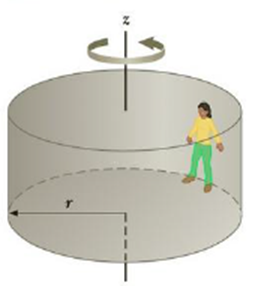Chapter 7, Problem 73AP

Chapter
Section
Textbook Problem

In a popular amusement park ride, a rotating cylinder of radius 3.00 m is set in rotation at an angular speed of 5.00 rad/s, as in Figure P7.73. The floor then drops away, leasing the riders suspended against the wall in a vertical position. What minimum coefficient of friction between a rider’s clothing and the wall is needed to keep the rider from slipping? Hint: Recall that the magnitude of the maximum force of static friction is equal to μsn, where n is the normal force—in this case, the force causing the centripetal acceleration.Figure P7.73

To determine
The minimum co-efficient of friction.

Explanation

Given info: Radius of the cylinder (r) is 3.00 m. The angular speed of rotation ( ω ) is 5.00 rad/s.

Explanation:

The normal force provides the centripetal acceleration. Therefore,

N=mω2r

• m is the mass of the person.

The maximum static force of friction equals the weight of the person. Hence,

fs=mg

• fs is the force of friction.
• g is the acceleration due to gravity.

The force of friction is,

fs=μsN

From the above equations,

mg=μsmω2r

On Re-arrang

Still sussing out bartleby?

Check out a sample textbook solution.

See a sample solution

The Solution to Your Study Problems

Bartleby provides explanations to thousands of textbook problems written by our experts, many with advanced degrees!

Get Started

When consumers guess at the calorie values in restaurant food portions, they generally overestimate. T F

Nutrition: Concepts and Controversies - Standalone book (MindTap Course List)

What is physically exchanged during crossing over?

Human Heredity: Principles and Issues (MindTap Course List)

What is the role of the cytochromes in the electron transport chain?

Chemistry for Today: General, Organic, and Biochemistry